## 5.3.1 Gate-Delay Time of CNT-FETs

The gate-delay time, which characterizes the switching response of the transistor, is an important metric for digital electronic applications. The gate-delay time of a transistor is defined as time taken to charge a constant gate capacitance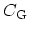to a voltage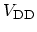at a constant current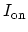(5.1)

The total gate capacitance is given by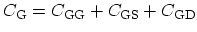, where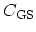and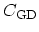are the gate-source and gate-drain parasitic capacitances, and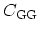can be written as, where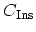is the gate insulator capacitance and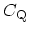is the so called quantum capacitance given by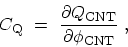(5.2)

where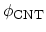is the electrostatic potential on the surface of the CNT and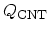is the total charge along the CNT. Given the one-dimensional density of states and assuming equilibrium conditions, (5.2) can be approximated as[265,264,266]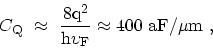(5.3)

where the twofold band and spin degeneracy is included. If thin and high-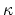insulators are used, then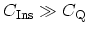and, implying that the potential on the CNT becomes equal to the gate potential (perfect coupling). This regime is called quantum capacitance limit in which the device is potential-controlled rather than charge-controlled . The insulator capacitance, occurring between the CNT and a cylindrical gate, is given by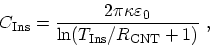(5.4)

where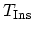is the gate insulator thickness and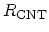is the radius of the CNT. Assuming a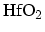gate insulator with a thickness of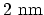,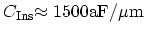, satisfying the condition of the quantum capacitance limit (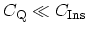). Parasitic capacitances are usually much larger than the quantum capacitance (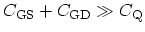) [268,269]. Therefore, the gate capacitance can be approximated as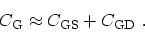(5.5)

M. Pourfath: Numerical Study of Quantum Transport in Carbon Nanotube-Based Transistors